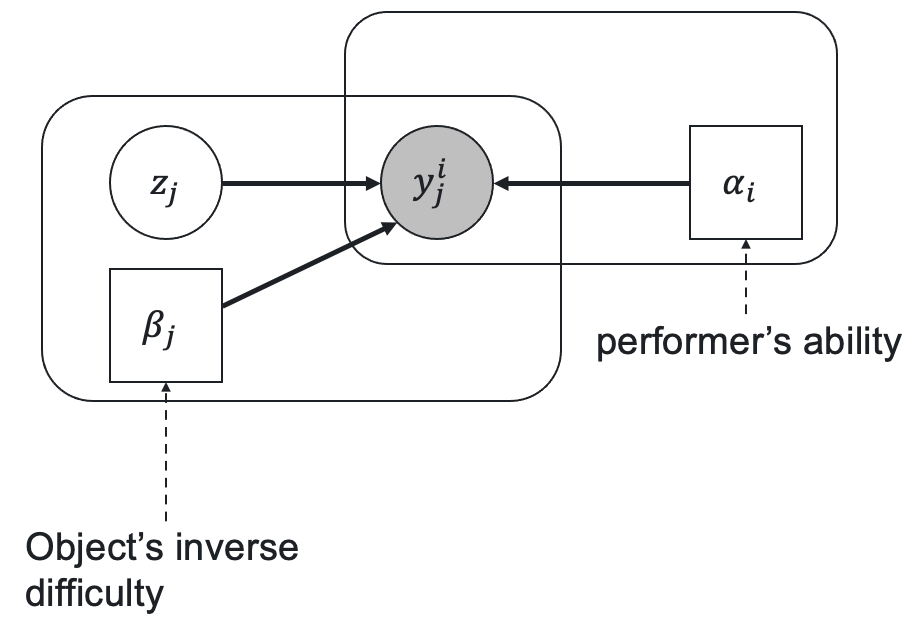• Overview
• Aggregations
• Aggregation
• Classification
• Embeddings
• Image segmentation
• SegmentationEM
• SegmentationMajorityVote
• SegmentationRASA
• Pairwise
• Texts
• ROVER
• TextHRRASA
• TextRASA
• TextSummarization
• utils
• Datasets
• Metrics
• Postprocessing

crowdkit.aggregation.classification.glad.GLAD | Source code

GLAD(
self,
n_iter: int = 100,
tol: float = 1e-05,
silent: bool = True,
labels_priors: Optional[Series] = None,
alphas_priors_mean: Optional[Series] = None,
betas_priors_mean: Optional[Series] = None,
m_step_max_iter: int = 25,
m_step_tol: float = 0.01
)


Generative model of Labels, Abilities, and Difficulties.

A probabilistic model that parametrizes workers' abilities and tasks' dificulties. Let's consider a case of $K$ class classification. Let $p$ be a vector of prior class probabilities, $\alpha_i \in (-\infty, +\infty)$ be a worker's ability parameter, $\beta_j \in (0, +\infty)$ be an inverse task's difficulty, $z_j$ be a latent variable representing the true task's label, and $y^i_j$ be a worker's response that we observe. The relationships between this variables and parameters according to GLAD are represented by the following latent label model:The prior probability of $z_j$ being equal to $c$ is

$\operatorname{Pr}(z_j = c) = p[c],$

the probability distribution of the worker's responses conditioned by the true label value $c$ follows the single coin Dawid-Skene model where the true label probability is a sigmoid function of the product of worker's ability and inverse task's difficulty:

$\operatorname{Pr}(y^i_j = k | z_j = c) = \begin{cases}a(i, j), & k = c \\ \frac{1 - a(i,j)}{K-1}, & k \neq c\end{cases},$

where

$a(i,j) = \frac{1}{1 + \exp(-\alpha_i\beta_j)}.$

Parameters $p$, $\alpha$, $\beta$ and latent variables $z$ are optimized through the Expectation-Minimization algorithm.

J. Whitehill, P. Ruvolo, T. Wu, J. Bergsma, and J. Movellan. Whose Vote Should Count More: Optimal Integration of Labels from Labelers of Unknown Expertise. Proceedings of the 22nd International Conference on Neural Information Processing Systems, 2009

https://proceedings.neurips.cc/paper/2009/file/f899139df5e1059396431415e770c6dd-Paper.pdf

## Parameters Description

Parameters Type Description
max_iter -

Maximum number of EM iterations.

eps -

Threshold for convergence criterion.

silent bool

If false, show progress bar.

labels_priors Optional[Series]

Prior label probabilities.

alphas_priors_mean Optional[Series]

Prior mean value of alpha parameters.

betas_priors_mean Optional[Series]

Prior mean value of beta parameters.

m_step_max_iter int

Maximum number of iterations of conjugate gradient method in M-step.

m_step_tol float

Tol parameter of conjugate gradient method in M-step.

labels_ Optional[Series]

Tasks' labels. A pandas.Series indexed by task such that labels.loc[task] is the tasks's most likely true label.

probas_ Optional[DataFrame]

Tasks' label probability distributions. A pandas.DataFrame indexed by task such that result.loc[task, label] is the probability of task's true label to be equal to label. Each probability is between 0 and 1, all task's probabilities should sum up to 1

alphas_ Series

workers' alpha parameters. A pandas.Series indexed by worker that contains estimated alpha parameters.

betas_ Series

Tasks' beta parameters. A pandas.Series indexed by task that contains estimated beta parameters.

Examples:

from crowdkit.aggregation import GLAD